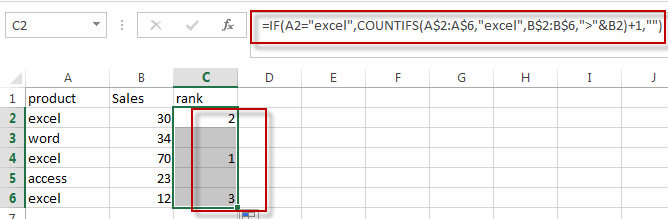# Rank values in a column based a specific value in another column

This post will guide you how to rank values in a column based on a specific value in another column in excel. How do I rank values in a range by a specific value in another column in excel.

## Rank Values Based on a Specific Value

Assuming that you have a list of data contains two columns and the first column is product list and another is Sales number. You want to rank the sales number of a specified product name, and you are not able to use the RANK function, because this function is only used to rank a value in a range. So how to achieve it.

You can try to write a complex formula based on the IF function and the COUNTIFS function to achieve the result.

For example, you want to rank values in range B2:B6 based on a specified value “excel” in the range A2:A6, then you can use the following formula:

`=IF(A2="excel",COUNTIFS(A\$2:A\$6,"excel",B\$2:B\$6,">"&B2)+1,"")`

Type this formula in another blank cell and then press Enter key in your keyboard, and drag the AutoFill Handler over other cells to apply this formula.You will see that the values in the range B2:B5 based on the “excel” in column A have been ranked.

### Related Functions

• Excel IF function
The Excel IF function perform a logical test to return one value if the condition is TRUE and return another value if the condition is FALSE. The IF function is a build-in function in Microsoft Excel and it is categorized as a Logical Function.The syntax of the IF function is as below:= IF (condition, [true_value], [false_value])….
• Excel Rank function
The Excel RANK function returns the rank of a given number in a supplied range of cells.  If there are duplicated values in a list of values, it will be set the same rank.The syntax of the RANK function is as below:= RANK (number,ref,[order])….
• Excel COUNTIFS function
The Excel COUNTIFS function returns the count of cells in a range that meet one or more criteria. The syntax of the COUNTIFS function is as below:= COUNTIFS(criteria_range1, criteria1, [criteria_range2, criteria2]…)…